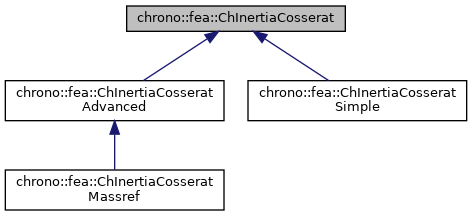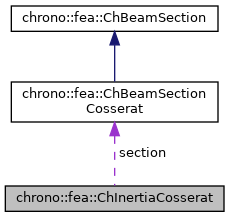chrono::fea::ChInertiaCosserat Class Referenceabstract

## Description

Base class for inertial properties (mass, moment of inertia) of beam sections of Cosserat type.

This can be shared between multiple beams.

#include <ChBeamSectionCosserat.h>

Inheritance diagram for chrono::fea::ChInertiaCosserat:[legend]
Collaboration diagram for chrono::fea::ChInertiaCosserat:[legend]

## Public Member Functions

virtual void ComputeInertiaMatrix (ChMatrixNM< double, 6, 6 > &Mi)=0
Compute the 6x6 sectional inertia matrix, as in {x_momentum,w_momentum}=[Mi]{xvel,wvel} The matrix is computed in the material reference (i.e. More...

virtual void ComputeInertiaDampingMatrix (ChMatrixNM< double, 6, 6 > &Ri, const ChVector<> &mW)
Compute the 6x6 sectional inertia damping matrix [Ri] (gyroscopic matrix damping), as in linearization dFi=[Mi]*d{xacc,wacc}+[Ri]*d{xvel,wvel}+[Ki]*d{pos,rot} The matrix is computed in the material reference, i.e. More...

virtual void ComputeInertiaStiffnessMatrix (ChMatrixNM< double, 6, 6 > &Ki, const ChVector<> &mWvel, const ChVector<> &mWacc, const ChVector<> &mXacc)
Compute the 6x6 sectional inertia stiffness matrix [Ki^], as in linearization dFi=[Mi]*d{xacc,wacc}+[Ri]*d{xvel,wvel}+[Ki]*d{pos,rot} The matrix is computed in the material reference. More...

virtual void ComputeQuadraticTerms (ChVector<> &mF, ChVector<> &mT, const ChVector<> &mW)=0
Compute the values of inertial force & torque depending on quadratic velocity terms, that is the gyroscopic torque and the centrifugal term (if any). More...

virtual void ComputeInertialForce (ChVector<> &mFi, ChVector<> &mTi, const ChVector<> &mWvel, const ChVector<> &mWacc, const ChVector<> &mXacc)
Compute the total inertial wrench, ie forces and torques (per unit length). More...

virtual double GetMassPerUnitLength ()=0
Compute mass per unit length, ex.SI units [kg/m]. More...

## Public Attributes

ChBeamSectionCosseratsection

bool compute_inertia_damping_matrix = true
Flag that turns on/off the computation of the [Ri] 'gyroscopic' inertial damping matrix. More...

bool compute_inertia_stiffness_matrix = true
Flag that turns on/off the computation of the [Ki] inertial stiffness matrix. More...

bool compute_Ri_Ki_by_num_diff = false
Flag for computing the Ri and Ki matrices via numerical differentiation even if an analytical expression is provided. More...

## Member Function Documentation

 void chrono::fea::ChInertiaCosserat::ComputeInertiaDampingMatrix ( ChMatrixNM< double, 6, 6 > & Ri, const ChVector<> & mW )
virtual

Compute the 6x6 sectional inertia damping matrix [Ri] (gyroscopic matrix damping), as in linearization dFi=[Mi]*d{xacc,wacc}+[Ri]*d{xvel,wvel}+[Ki]*d{pos,rot} The matrix is computed in the material reference, i.e.

both linear and rotational coords assumed in the basis of the centerline reference. Default implementation: falls back to numerical differentiation of ComputeInertialForce to compute Ri, please override this if analytical formula of Ri is known!

Parameters
 Ri 6x6 sectional inertial-damping (gyroscopic damping) matrix here mW current angular velocity of section, in material frame

## ◆ ComputeInertialForce()

 void chrono::fea::ChInertiaCosserat::ComputeInertialForce ( ChVector<> & mFi, ChVector<> & mTi, const ChVector<> & mWvel, const ChVector<> & mWacc, const ChVector<> & mXacc )
virtual

Compute the total inertial wrench, ie forces and torques (per unit length).

Note: both force and torque are returned in the basis of the material frame (not the absolute frame!), ex. to apply it to a Chrono body, the force must be rotated to absolute basis. Default implementation: falls back to Fi = [Mi]*{xacc,wacc}+{mF,mT} where [Mi] is given by ComputeInertiaMatrix() and {F_quad,T_quad} are given by ComputeQuadraticTerms(), i.e. gyro and centrif.terms. For faster implementations one can override this, ex. avoid doing the [Mi] matrix product.

Parameters
 mFi total inertial force returned here, in basis of material frame mTi total inertial torque returned here, in basis of material frame mWvel current angular velocity of section, in material frame mWacc current angular acceleration of section, in material frame mXacc current acceleration of section, in material frame (not absolute!)

## ◆ ComputeInertiaMatrix()

 virtual void chrono::fea::ChInertiaCosserat::ComputeInertiaMatrix ( ChMatrixNM< double, 6, 6 > & Mi )
pure virtual

Compute the 6x6 sectional inertia matrix, as in {x_momentum,w_momentum}=[Mi]{xvel,wvel} The matrix is computed in the material reference (i.e.

it is the sectional mass matrix)

Parameters
 Mi 6x6 sectional mass matrix here

## ◆ ComputeInertiaStiffnessMatrix()

 void chrono::fea::ChInertiaCosserat::ComputeInertiaStiffnessMatrix ( ChMatrixNM< double, 6, 6 > & Ki, const ChVector<> & mWvel, const ChVector<> & mWacc, const ChVector<> & mXacc )
virtual

Compute the 6x6 sectional inertia stiffness matrix [Ki^], as in linearization dFi=[Mi]*d{xacc,wacc}+[Ri]*d{xvel,wvel}+[Ki]*d{pos,rot} The matrix is computed in the material reference.

NOTE the matrix already contains the 'geometric' stiffness, so it transforms to absolute transl/local rot just like [Mi] and [Ri]: [Ki]_al =[R,0;0,I]*[Ki^]*[R',0;0,I'] , with [Ki^]=([Ki]+[0,f~';0,0]) for f=current force part of inertial forces. Default implementation: falls back to numerical differentiation of ComputeInertialForce to compute Ki^, please override this if analytical formula of Ki^ is known!

Parameters
 Ki 6x6 sectional inertial-stiffness matrix [Ki^] here mWvel current angular velocity of section, in material frame mWacc current angular acceleration of section, in material frame mXacc current acceleration of section, in material frame (not absolute!)

 virtual void chrono::fea::ChInertiaCosserat::ComputeQuadraticTerms ( ChVector<> & mF, ChVector<> & mT, const ChVector<> & mW )
pure virtual

Compute the values of inertial force & torque depending on quadratic velocity terms, that is the gyroscopic torque and the centrifugal term (if any).

All terms expressed in the material reference, ie. the reference in the centerline of the section.

Parameters
 mF centrifugal term (if any) returned here mT gyroscopic term returned here mW current angular velocity of section, in material frame

## ◆ GetMassPerUnitLength()

 virtual double chrono::fea::ChInertiaCosserat::GetMassPerUnitLength ( )
pure virtual

Compute mass per unit length, ex.SI units [kg/m].

This is also the(0, 0) element in the sectional inertia matrix.

## ◆ compute_inertia_damping_matrix

 bool chrono::fea::ChInertiaCosserat::compute_inertia_damping_matrix = true

Flag that turns on/off the computation of the [Ri] 'gyroscopic' inertial damping matrix.

If false, Ri=0. Can be used for cpu speedup, profiling, tests. Default: true.

## ◆ compute_inertia_stiffness_matrix

 bool chrono::fea::ChInertiaCosserat::compute_inertia_stiffness_matrix = true

Flag that turns on/off the computation of the [Ki] inertial stiffness matrix.

If false, Ki=0. Can be used for cpu speedup, profiling, tests. Default: true.

## ◆ compute_Ri_Ki_by_num_diff

 bool chrono::fea::ChInertiaCosserat::compute_Ri_Ki_by_num_diff = false

Flag for computing the Ri and Ki matrices via numerical differentiation even if an analytical expression is provided.

Children calsses must take care of this. Default: false.

The documentation for this class was generated from the following files:
• /builds/uwsbel/chrono/src/chrono/fea/ChBeamSectionCosserat.h
• /builds/uwsbel/chrono/src/chrono/fea/ChBeamSectionCosserat.cpp